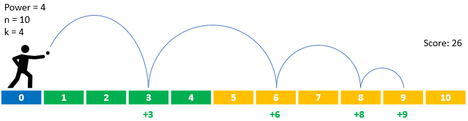# One Ball Many Chocolates

Limits 1s, 512 MB

Alice, Bob and Charlie, team ABC have come to the venue of world finals to be inspired by the world finalists.

For the entertainment purpose of the contestants and the visitors, some game segments have been set up. One such game has taken the full attention of the team because of the prize. The prize is that the participant will get $p$ chocolates if he manages to score $p$ points in the game and all the members of team ABC love chocolates.

The game is very simple. You have to throw a ball not more than once.

Well, there are some more details.

There will be $\bf{n}$ blocks numbered from $\bf{1}$ to $\bf{n}$ and their lengths are $\bf{1}$ unit. You will have to stand before the first block and throw the ball on a block whose number is at most $\bf{k}$.

You will get $\bf{i}$ points if the ball lands on block $\bf{i}$. When the ball is thrown, you can make it bounce and land in multiple blocks to gain more points. Initially you have $\bf{0}$. It’s possible that the ball can move out of the play area but as there aren’t any blocks there no more scores would be added.

To make the ball bounce, you have to throw the ball with a certain power level. With each bounce, the ball will lose $\bf{1}$ power, thus will move $\bf{1}$ unit less than the previous bounce.

So, let’s say you are playing the game where $n=10$ and $k=4$ you have thrown the ball with power $4$ on the $3^{rd}$ block as it’s number is less than $k$.

First drop will be on the $3^{rd}$ block with power $4$.
After bouncing on $3^{rd}$ block, power = $3$, score = $3$, next block = $6^{th}$
After bouncing on $6^{th}$ block, power = $2$, score = $9$, next block = $8^{th}$
After bouncing on $8^{th}$ block, power = $1$, score = $17$, next block = $9^{th}$
After bouncing on $9^{th}$ block, power = $0$, score = $26$, next block = having no power, none.

This is just an example. There might be a more optimal solution.Now, you are not playing the game. But you can see Team ABC’s interesting approach to gain the maximum amount of points. Alice is thinking of a clever solution to gain the most points. Bob on the other hand, started to calculate all possible outcomes for all possible actions to find the most winning strategy, But Charlie has thrown the ball and won the maximum amount of points and the maximum number of chocolates one can get from that game. The announcer specially announced this incident but didn’t disclose how many points Charlie acquired. As Charlie and the team have already won a huge amount of chocolates, they stopped investing their time on this game and moved on to the next one.

You as an observant one, want to find out the exact points Charlie earned, as you also love chocolates. You already realized that having more power always won't do you any good, so you will never throw the ball with power more than $\bf{n}$.

Given $\bf{n}$ and $\bf{k}$, you have to find out the maximum score(chocolates) one can get from the game.

## Input

The first and only line of input will contain 2 space separated integers $\bf{n}$ and $\bf{k}$.

$1 \le k \le n \le 10000$

## Output

You have to output an integer which is the maximum number of chocolates you can gain from the game.

## Samples

InputOutput
10 5

30


Throwing the ball on the $4^{th}$ block with power $4$ will yield the maximum score.

InputOutput
28 5

126


Throwing the ball on the $5^{th}$ block with power $7$ will yield the maximum score.